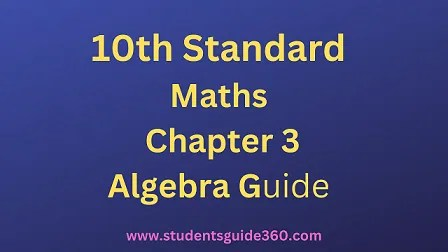# 10th Maths Chapter 3 Algebra Exercise 3.14

10th Standard Maths Chapter 3 Algebra Samacheer Kalvi Guide Exercise 3.14 Book Back Answers Solutions. TN 10th SSLC Samacheer Kalvi Guide. 10th All Subject Guide – Click Here. Class 1 to 12 All Subject Book Back Answers – Click Here## 10th Maths Chapter 3 Algebra Exercise 3.14

### 1. Write each of the following expressions in terms of α + β and αβ.Solution:### 2.The roots of the equation 2×2 – 7x + 5 = 0 are α and β. Without solving the root findSolution:
2×2 – 7x + 5 = x2 – 72x+52 = 0
α + β = 72
αβ = 52### 3.The roots of the equation x2 + 6x – 4 = 0 are α, β. Find the quadratic equation whose roots are

• (i) α2 and β2
• (ii) 2α and 2β
• (iii) α2β and β2α

Solution:
If the roots are given, the quadratic equation is x2 – (sum of the roots) x + product the roots = 0.
For the given equation.
x2 + 6x – 4 = 0
α + β = -6
αβ = -4
(i) α2 + β2 = (α + β)2 – 2αβ
= (-6)2 – 2(-4) = 36 + 8 = 44
α2β2 = (αβ)2 = (-4)2 = 16
∴ The required equation is x2 – 44x – 16 = 0.(iii) α2β + β2α = αβ(α + β)
= -4(-6) = 24
α2β × β2α = α3β3 = (αβ)3 = (-4)3 = -64
∴ The required equation = x2 – 24x – 64 – 0.

### 4.If α, β are the roots of 7×2 + ax + 2 = 0 and if β – α = −13/7 Find the values of a.

Solution:### 5. If one root of the equation 2y2 – ay + 64 = 0 is twice the other then find the values of a.

Solution:
Let one of the root α = 2β
α + β = 2β + β = 3β
Givena2 = 576
a = 24, -24

### 6. If one root of the equation 3×2 + kx + 81 = 0 (having real roots) is the square of the other then find k.

Solution:
3×2 + kx + 81 = 0
Let the roots be α and α2